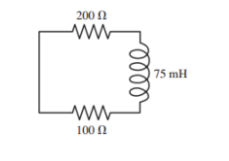# Problem: At t =0s, the current in the circuit in the figure is I0. At what time is the current 12I0?

###### FREE Expert Solution

Current:

$\overline{){\mathbf{I}}{\mathbf{\left(}}{\mathbf{t}}{\mathbf{\right)}}{\mathbf{=}}{{\mathbf{I}}}_{{\mathbf{0}}}{{\mathbf{e}}}^{\mathbf{-}\mathbf{t}}{\mathbf{T}}}}$

83% (153 ratings)###### Problem Details

At t =0s, the current in the circuit in the figure is I0. At what time is the current 12I0?Frequently Asked Questions

What scientific concept do you need to know in order to solve this problem?

Our tutors have indicated that to solve this problem you will need to apply the Series LRC Circuits concept. You can view video lessons to learn Series LRC Circuits. Or if you need more Series LRC Circuits practice, you can also practice Series LRC Circuits practice problems.

What professor is this problem relevant for?

Based on our data, we think this problem is relevant for Professor Hanna's class at PSU.